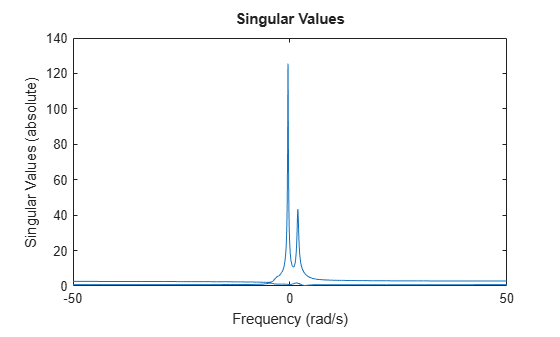# getPeakGain

Peak gain of dynamic system frequency response

## Syntax

``gpeak = getPeakGain(sys)``
``gpeak = getPeakGain(sys,tol)``
``gpeak = getPeakGain(sys,tol,fband)``
``````[gpeak,fpeak] = getPeakGain(___)``````

## Description

example

````gpeak = getPeakGain(sys)` returns the peak input/output gain in absolute units of the dynamic system model, `sys`.If `sys` is a SISO model, then the peak gain is the largest value of the frequency response magnitude. If `sys` is a MIMO model, then the peak gain is the largest value of the frequency response 2-norm (the largest singular value across frequency) of `sys`. This quantity is also called the L∞ norm of `sys`, and coincides with the H∞ norm for stable systems (see `norm`).If `sys` is a model that has tunable or uncertain parameters, `getPeakGain` evaluates the peak gain at the current or nominal value of `sys`.If `sys` is a model array, `getPeakGain` returns an array of the same size as `sys`, where ```gpeak(k) = getPeakGain(sys(:,:,k))``` .```

example

````gpeak = getPeakGain(sys,tol)` returns the peak gain of `sys` with relative accuracy `tol`. ```

example

````gpeak = getPeakGain(sys,tol,fband)` returns the peak gain in the frequency interval `fband` = `[fmin,fmax]` with 0 ≤ `fmin` < `fmax`. This syntax also takes into account the negative frequencies in the band `[–fmax,–fmin]` for models with complex coefficients.```

example

``````[gpeak,fpeak] = getPeakGain(___)``` also returns the frequency `fpeak` at which the gain achieves the peak value `gpeak`, and can include any of the input arguments in previous syntaxes. `fpeak` can be negative for systems with complex coefficients.```

## Examples

collapse all

Compute the peak gain of the resonance in the following transfer function:

`$sys=\frac{90}{{s}^{2}+1.5s+90}.$`

```sys = tf(90,[1,1.5,90]); gpeak = getPeakGain(sys)```
```gpeak = 6.3444 ```

The `getPeakGain` command returns the peak gain in absolute units.

Compute the peak gain of the resonance in the transfer function with a relative accuracy of 0.01%.

`$sys=\frac{90}{{s}^{2}+1.5s+90}.$`

```sys = tf(90,[1,1.5,90]); gpeak = getPeakGain(sys,0.0001)```
```gpeak = 6.3444 ```

The second argument specifies a relative accuracy of 0.0001. The `getPeakGain` command returns a value that is within 0.0001 (0.01%) of the true peak gain of the transfer function. By default, the relative accuracy is 0.01 (1%).

Compute the peak gain of the higher-frequency resonance in the transfer function

`$sys=\left(\frac{1}{{s}^{2}+0.2s+1}\right)\left(\frac{100}{{s}^{2}+s+100}\right).$`

`sys` is the product of resonances at 1 rad/s and 10 rad/s.

```sys = tf(1,[1,.2,1])*tf(100,[1,1,100]); fband = [8,12]; gpeak = getPeakGain(sys,0.01,fband);```

The `fband` argument causes `getPeakGain` to return the local peak gain between 8 and 12 rad/s.

Identify which of the two resonances has higher gain in the transfer function

`$sys=\left(\frac{1}{{s}^{2}+0.2s+1}\right)\left(\frac{100}{{s}^{2}+s+100}\right).$`

`sys` is the product of resonances at 1 rad/s and 10 rad/s.

```sys = tf(1,[1,.2,1])*tf(100,[1,1,100]); [gpeak,fpeak] = getPeakGain(sys)```
```gpeak = 5.0747 ```
```fpeak = 0.9902 ```

`fpeak` is the frequency corresponding to the peak gain `gpeak`. The peak at 1 rad/s is the overall peak gain of `sys`.

For systems with complex coefficients, `getPeakGain` can return a peak at a negative or positive frequency depending on the shape and `fband` you specify.

Generate a random state-space model with complex data.

```rng(1) A = complex(randn(10),randn(10)); B = complex(randn(10,3),randn(10,3)); C = complex(randn(2,10),randn(2,10)); D = complex(randn(2,3),randn(2,3)); sys = ss(A,B,C,D);```

Compute the peak gain with a relative accuracy of 0.1%. Also, specify `fband` = `[0,1]` to compute the peak in the frequency interval [–1,1].

`[gPeak,fPeak] = getPeakGain(sys,1e-3,[0,1])`
```gPeak = 126.2396 ```
```fPeak = -0.4579 ```

In this interval, `sys` attains a peak at a negative frequency value. Create a singular value plot in this range to confirm the result.

```w = linspace(-1,1,100); opt = sigmaoptions; opt.FreqScale = 'Linear'; opt.MagUnits = 'abs'; sigmaplot(sys,w,opt)```Now compute the peak gain in the frequency interval [–50,–1] ∪ [1,50]. To do so, specify `fband` = `[1,50]`.

`[gPeak,fPeak] = getPeakGain(sys,1e-3,[1,50])`
```gPeak = 43.3303 ```
```fPeak = 1.8097 ```

In this interval, `sys` attains a peak at a positive frequency value.

Create a singular value plot in this range to confirm the result.

```w = linspace(-50,50,5000); sigmaplot(sys,w,opt)```For this interval, `getPeakGain` returns the magnitude and frequency value for the smaller peak shown in the plot.

## Input Arguments

collapse all

Input dynamic system, specified as any dynamic system model or model array. `sys` can be SISO or MIMO.

Relative accuracy of the peak gain, specified as a positive real scalar value. `getPeakGain` calculates `gpeak` such that the fractional difference between `gpeak` and the true peak gain of `sys` is no greater than `tol`. The default value is 0.01, meaning that `gpeak` is within 1% of the true peak gain.

Frequency interval in which to calculate the peak gain, specified as a 1-by-2 vector of positive real values. Specify `fband` as a row vector of the form `[fmin,fmax]` with 0 ≤ `fmin` < `fmax`.

For models with complex coefficients, `getPeakGain` calculates the peak gain in the range `[–fmax,–fmin]``[fmin,fmax]`. As a result, the function can return a peak at a negative frequency.

## Output Arguments

collapse all

Peak gain of the dynamic system model or model array `sys`, returned as a scalar value or an array.

• If `sys` is a single model, then `gpeak` is a scalar value.

• If `sys` is a model array, then `gpeak` is an array of the same size as `sys`, where ```gpeak(k) = getPeakGain(sys(:,:,k))```.

Frequency at which the gain achieves the peak value `gpeak`, returned as a real scalar value or an array of real values. The frequency is expressed in units of rad/`TimeUnit`, relative to the `TimeUnit` property of `sys`.

• If `sys` is a single model, then `fpeak` is a scalar.

• If `sys` is a model array, then `fpeak` is an array of the same size as `sys`, where `fpeak(k)` is the peak gain frequency of `sys(:,:,k)`.

`fpeak` can be negative for systems with complex coefficients.

## Algorithms

`getPeakGain` uses the algorithm of . All eigenvalue computations are performed using structure-preserving algorithms from the SLICOT library. For more information about the SLICOT library, see http://slicot.org.

 Bruinsma, N.A., and M. Steinbuch. "A Fast Algorithm to Compute the H Norm of a Transfer Function Matrix." Systems & Control Letters, 14, no.4 (April 1990): 287–93.